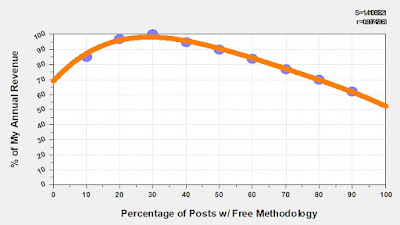## March 08, 2023

### Odd Quirks in Category Targeting Models

Here's one of the odd quirks you find when creating Category Targeting Models. This brand has twelve (12) main categories. When I decile my findings, I see oddities. Look at this customer (decile 10 is best, decile 1 is worst).

• Category 01 = 10.
• Category 02 = 10.
• Category 03 = 10.
• Category 04 = 8.
• Category 05 = 10.
• Category 06 = 10.
• Category 07 = 10.
• Category 08 = 10.
• Category 09 = 10.
• Category 10 = 10.
• Category 11 = 10.
• Category 12 = 10.
You'd want to target this customer with EVERY category, correct? Yeah! What's interesting is that this customer possesses weighted dollars within Categories 1/6/7/8/9/10/11. That's a really good customer. And all of that cross-shopping suggests that the customer would also be a prime candidate for Categories 2/3/4/5/12, even with no prior purchase history in those categories.

This is not an uncommon outcome.

Your best customers should be exposed to EVERYTHING you sell, period.

It's the marginal customers where targeting makes a huge difference. Look at this customer.

• Category 01 = 2.
• Category 02 = 2.
• Category 03 = 3.
• Category 04 = 4.
• Category 05 = 2.
• Category 06 = 4.
• Category 07 = 4.
• Category 08 = 2.
• Category 09 = 2.
• Category 10 = 7.
• Category 11 = 2.
• Category 12 = 2.
There you go ... target that customer with Category 10, right?

Each brand possesses some odd quirks in their Category Targeting Models. Figure out what those quirks are, and when you are targeting your customers take advantage of the odd behaviors you observe.